Eric Smith

Systems of Inequalities

Slide Duration:

Section 1: Properties of Real Numbers
Basic Types of Numbers

30m 41s

Intro
0:00
Objectives
0:07
Basic Types of Numbers
0:36
Natural Numbers
1:02
Whole Numbers
1:29
Integers
2:04
Rational Numbers
2:38
Irrational Numbers
5:06
Imaginary Numbers
6:48
Basic Types of Numbers Cont.
8:09
The Big Picture
8:10
Real vs. Imaginary Numbers
8:30
Rational vs. Irrational Numbers
8:48
Basic Types of Numbers Cont.
10:55
Number Line
11:06
Absolute Value
11:44
Inequalities
12:39
Example 1
13:16
Example 2
17:30
Example 3
21:56
Example 4
24:27
Example 5
27:48
Operations on Numbers

19m 26s

Intro
0:00
Objectives
0:06
Operations on Numbers
0:25
0:53
Subtraction
1:33
Multiplication & Division
2:19
Exponents
3:24
Bases
4:04
Square Roots
4:59
Principle Square Roots
5:09
Perfect Squares
6:32
Simplifying and Combining Roots
6:52
Example 1
8:16
Example 2
12:30
Example 3
14:02
Example 4
16:27
Order of Operations

12m 6s

Intro
0:00
Objectives
0:06
The Order of Operations
0:25
Work Inside Parentheses
0:42
Simplify Exponents
0:52
Multiplication & Division from Left to Right
0:57
Addition & Subtraction from Left to Right
1:11
Remember PEMDAS
1:21
The Order of Operations Cont.
2:27
Example
2:43
Example 1
3:55
Example 2
5:36
Example 3
7:35
Example 4
8:56
Properties of Real Numbers

18m 52s

Intro
0:00
Objectives
0:07
The Properties of Real Numbers
0:23
Commutative Property of Addition and Multiplication
0:44
Associative Property of Addition and Multiplication
1:50
Distributive Property of Multiplication Over Addition
3:20
Division Property of Zero
4:46
Division Property of One
5:23
Multiplication Property of Zero
5:56
Multiplication Property of One
6:17
6:29
Why Are These Properties Important?
6:53
Example 1
9:16
Example 2
13:04
Example 3
14:30
Example 4
16:57
Section 2: Linear Equations
The Vocabulary of Linear Equations

12m 22s

Intro
0:00
Objectives
0:09
The Vocabulary of Linear Equations
0:44
Variables
0:52
Terms
1:09
Coefficients
1:40
Like Terms
2:18
Examples of Like Terms
2:37
Expressions
4:01
Equations
4:26
Linear Equations
5:04
Solutions
5:55
Example 1
6:16
Example 2
7:16
Example 3
8:45
Example 4
10:20
Solving Linear Equations in One Variable

28m 52s

Intro
0:00
Objectives
0:08
Solving Linear Equations in One Variable
0:34
Conditional Cases
0:51
Identity Cases
1:09
1:30
Solving Linear Equations in One Variable Cont.
2:00
2:10
Multiplication Property of Equality
2:43
Steps to Solve Linear Equations
3:14
Example 1
4:22
Example 2
8:21
Example 3
12:32
Example 4
14:19
Example 5
17:25
Example 6
22:17
Solving Formulas

12m 2s

Intro
0:00
Objectives
0:06
Solving Formulas
0:18
Formulas
0:26
Use the Same Properties as Solving Linear Equations
1:36
1:55
Multiplication Property of Equality
1:58
Steps to Solve Formulas
2:43
Example 1
3:56
Example 2
6:09
Example 3
8:39
Applications of Linear Equations

28m 41s

Intro
0:00
Objectives
0:10
Applications of Linear Equations
0:43
The Six-Step Method to Solving Word Problems
0:55
Common Terms
3:12
Example 1
5:03
Example 2
9:40
Example 3
13:48
Example 4
17:58
Example 5
23:28
Applications of Linear Equations, Motion & Mixtures

24m 26s

Intro
0:00
Objectives
0:21
Motion and Mixtures
0:46
Motion Problems: Distance, Rate, and Time
1:06
Mixture Problems: Amount, Percent, and Total
1:27
The Table Method
1:58
The Beaker Method
3:38
Example 1
5:05
Example 2
9:44
Example 3
14:20
Example 4
19:13
Section 3: Graphing
Rectangular Coordinate System

22m 55s

Intro
0:00
Objectives
0:11
The Rectangular Coordinate System
0:39
The Cartesian Coordinate System
0:40
X-Axis
0:54
Y-Axis
1:04
Origin
1:11
1:26
Ordered Pairs
2:10
Example 1
2:55
The Rectangular Coordinate System Cont.
6:09
X-Intercept
6:45
Y-Intercept
6:55
Relation of X-Values and Y-Values
7:30
Example 2
11:03
Example 3
12:13
Example 4
14:10
Example 5
18:38
Slope & Graphing

27m 58s

Intro
0:00
Objectives
0:11
Slope and Graphing
0:48
Standard Form
1:14
Example 1
2:24
Slope and Graphing Cont.
4:58
Slope, m
5:07
Slope is Rise over Run
6:11
Don't Mix Up the Coordinates
8:20
Example 2
9:39
Slope and Graphing Cont.
14:26
Slope-Intercept Form
14:34
Example 3
16:55
Example 4
18:00
Slope and Graphing Cont.
19:00
Rewriting an Equation in Slope-Intercept Form
19:39
Rewriting an Equation in Standard Form
20:09
Slopes of Vertical & Horizontal Lines
20:56
Example 5
22:49
Example 6
24:09
Example 7
25:59
Example 8
26:57
Linear Equations in Two Variables

20m 36s

Intro
0:00
Objectives
0:13
Linear Equations in Two Variables
0:36
Point-Slope Form
1:07
Substitute in the Point and the Slope
2:21
Parallel Lines: Two Lines with the Same Slope
4:05
Perpendicular Lines: Slopes are Negative Reciprocals of Each Other
4:39
Perpendicular Lines: Product of Slopes is -1
5:24
Example 1
6:02
Example 2
7:50
Example 3
10:49
Example 4
13:26
Example 5
15:30
Example 6
17:43
Section 4: Functions
Introduction to Functions

21m 24s

Intro
0:00
Objectives
0:07
Introduction to Functions
0:58
Relations
1:03
Functions
1:37
Independent Variables
2:00
Dependent Variables
2:11
Function Notation
2:21
Function
3:43
Input and Output
3:53
Introduction to Functions Cont.
4:45
Domain
4:46
Range
4:55
Functions Represented by a Diagram
6:41
Natural Domain
9:11
Evaluating Functions
12:02
Example 1
13:13
Example 2
15:03
Example 3
16:18
Example 4
19:54
Graphing Functions

16m 12s

Intro
0:00
Objectives
0:09
Graphing Functions
0:54
Using Slope-Intercept Form
1:56
Vertical Line Test
2:58
Determining the Domain
4:20
Determining the Range
5:43
Example 1
6:06
Example 2
7:18
Example 3
8:31
Example 4
11:04
Section 5: Systems of Linear Equations
Systems of Linear Equations

25m 54s

Intro
0:00
Objectives
0:13
Systems of Linear Equations
0:46
System of Equations
0:51
System of Linear Equations
1:15
Solutions
1:35
Points as Solutions
1:53
Finding Solutions Graphically
5:13
Example 1
6:37
Example 2
12:07
Systems of Linear Equations Cont.
17:01
One Solution, No Solution, or Infinite Solutions
17:10
Example 3
18:31
Example 4
22:37
Solving a System Using Substitution

20m 1s

Intro
0:00
Objectives
0:09
Solving a System Using Substitution
0:32
Substitution Method
1:24
Substitution Example
2:35
One Solution, No Solution, or Infinite Solutions
7:50
Example 1
9:45
Example 2
12:48
Example 3
15:01
Example 4
17:30
Solving a System Using Elimination

19m 40s

Intro
0:00
Objectives
0:09
Solving a System Using Elimination
0:27
Elimination Method
0:42
Elimination Example
2:01
One Solution, No Solution, or Infinite Solutions
7:05
Example 1
8:53
Example 2
11:46
Example 3
15:37
Example 4
17:45
Applications of Systems of Equations

24m 34s

Intro
0:00
Objectives
0:12
Applications of Systems of Equations
0:30
Word Problems
1:31
Example 1
2:17
Example 2
7:55
Example 3
13:07
Example 4
17:15
Section 6: Inequalities
Solving Linear Inequalities in One Variable

17m 13s

Intro
0:00
Objectives
0:08
Solving Linear Inequalities in One Variable
0:37
Inequality Expressions
0:46
Linear Inequality Solution Notations
3:40
Inequalities
3:51
Interval Notation
4:04
Number Lines
4:43
Set Builder Notation
5:24
Use Same Techniques as Solving Equations
6:59
'Flip' the Sign when Multiplying or Dividing by a Negative Number
7:12
'Flip' Example
7:50
Example 1
8:54
Example 2
11:40
Example 3
14:01
Compound Inequalities

16m 13s

Intro
0:00
Objectives
0:07
Compound Inequalities
0:37
'And' vs. 'Or'
0:44
'And'
3:24
'Or'
3:35
'And' Symbol, or Intersection
3:51
'Or' Symbol, or Union
4:13
Inequalities
4:41
Example 1
6:22
Example 2
9:30
Example 3
11:27
Example 4
13:49
Solving Equations with Absolute Values

14m 12s

Intro
0:00
Objectives
0:08
Solve Equations with Absolute Values
0:18
Solve Equations with Absolute Values Cont.
1:11
Steps to Solving Equations with Absolute Values
2:21
Example 1
3:23
Example 2
6:34
Example 3
10:12
Inequalities with Absolute Values

17m 7s

Intro
0:00
Objectives
0:07
Inequalities with Absolute Values
0:23
Recall…
2:08
Example 1
3:39
Example 2
6:06
Example 3
8:14
Example 4
10:29
Example 5
13:29
Graphing Inequalities in Two Variables

15m 33s

Intro
0:00
Objectives
0:07
Graphing Inequalities in Two Variables
0:32
Split Graph into Two Regions
1:53
Graphing Inequalities
5:44
Test Points
6:20
Example 1
7:11
Example 2
10:17
Example 3
13:06
Systems of Inequalities

21m 13s

Intro
0:00
Objectives
0:08
Systems of Inequalities
0:24
Test Points
1:10
Steps to Solve Systems of Inequalities
1:25
Example 1
2:23
Example 2
7:28
Example 3
12:51
Section 7: Polynomials
Integer Exponents

44m 51s

Intro
0:00
Objectives
0:09
Integer Exponents
0:42
Exponents 'Package' Multiplication
1:25
Example 1
2:00
Example 2
3:13
Integer Exponents Cont.
4:50
Product Rule for Exponents
4:51
Example 3
7:16
Example 4
10:15
Integer Exponents Cont.
13:13
Power Rule for Exponents
13:14
Power Rule with Multiplication and Division
15:33
Example 5
16:18
Integer Exponents Cont.
20:04
Example 6
20:41
Integer Exponents Cont.
25:52
Zero Exponent Rule
25:53
Quotient Rule
28:24
Negative Exponents
30:14
Negative Exponent Rule
32:27
Example 7
34:05
Example 8
36:15
Example 9
39:33
Example 10
43:16

18m 33s

Intro
0:00
Objectives
0:07
0:25
Terms
0:33
Coefficients
0:51
1:13
Like Terms
1:29
Polynomials
2:21
Monomials, Binomials, Trinomials, and Polynomials
5:41
Degrees
7:00
Evaluating Polynomials
8:12
9:25
Example 1
11:48
Example 2
13:00
Example 3
14:41
Example 4
16:15
Multiplying Polynomials

25m 7s

Intro
0:00
Objectives
0:06
Multiplying Polynomials
0:41
Distributive Property
1:00
Example 1
2:49
Multiplying Polynomials Cont.
8:22
Organize Terms with a Table
8:23
Example 2
13:40
Multiplying Polynomials Cont.
16:33
Multiplying Binomials with FOIL
16:48
Example 3
18:49
Example 4
20:04
Example 5
21:42
Dividing Polynomials

44m 56s

Intro
0:00
Objectives
0:07
Dividing Polynomials
0:29
Dividing Polynomials by Monomials
2:10
Dividing Polynomials by Polynomials
2:59
Dividing Numbers
4:09
Dividing Polynomials Example
8:39
Example 1
12:35
Example 2
14:40
Example 3
16:45
Example 4
21:13
Example 5
24:33
Example 6
29:02
Dividing Polynomials with Synthetic Division Method
33:36
Example 7
38:43
Example 8
42:24
Section 8: Factoring Polynomials
Greatest Common Factor & Factor by Grouping

28m 27s

Intro
0:00
Objectives
0:09
Greatest Common Factor
0:31
Factoring
0:40
Greatest Common Factor (GCF)
1:48
GCF for Polynomials
3:28
Factoring Polynomials
6:45
Prime
8:21
Example 1
9:14
Factor by Grouping
14:30
Steps to Factor by Grouping
17:03
Example 2
17:43
Example 3
19:20
Example 4
20:41
Example 5
22:29
Example 6
26:11
Factoring Trinomials

21m 44s

Intro
0:00
Objectives
0:06
Factoring Trinomials
0:25
Recall FOIL
0:26
Factor a Trinomial by Reversing FOIL
1:52
Tips when Using Reverse FOIL
5:31
Example 1
7:04
Example 2
9:09
Example 3
11:15
Example 4
13:41
Factoring Trinomials Cont.
15:50
Example 5
18:42
Factoring Trinomials Using the AC Method

30m 9s

Intro
0:00
Objectives
0:08
Factoring Trinomials Using the AC Method
0:27
Factoring when Leading Term has Coefficient Other Than 1
1:07
Reversing FOIL
1:18
Example 1
1:46
Example 2
4:28
Factoring Trinomials Using the AC Method Cont.
7:45
The AC Method
8:03
Steps to Using the AC Method
8:19
Tips on Using the AC Method
9:29
Example 3
10:45
Example 4
16:50
Example 5
21:08
Example 6
24:58
Special Factoring Techniques

30m 14s

Intro
0:00
Objectives
0:07
Special Factoring Techniques
0:26
Difference of Squares
1:46
Perfect Square Trinomials
2:38
No Sum of Squares
3:32
Special Factoring Techniques Cont.
4:03
Difference of Squares Example
4:04
Perfect Square Trinomials Example
5:29
Example 1
7:31
Example 2
9:59
Example 3
11:47
Example 4
15:09
Special Factoring Techniques Cont.
19:07
Sum of Cubes and Difference of Cubes
19:08
Example 5
23:13
Example 6
26:12

23m 38s

Intro
0:00
Objectives
0:08
0:19
0:20
Zero Factor Property
1:39
Zero Factor Property Example
2:34
Example 1
4:00
Solving Quadratic Equations by Factoring Cont.
5:54
Example 2
7:28
Example 3
11:09
Example 4
14:22
Solving Quadratic Equations by Factoring Cont.
18:17
Higher Degree Polynomial Equations
18:18
Example 5
20:22

29m 27s

Intro
0:00
Objectives
0:12
0:29
Linear Factors
0:38
1:22
Principle of Square Roots
3:36
Completing the Square
4:50
Steps for Using Completing the Square
5:15
Completing the Square Works on All Quadratic Equations
6:41
7:28
Discriminants
8:25
10:11
Example 1
11:54
Example 2
13:03
Example 3
16:30
Example 4
21:29
Example 5
25:07

16m 47s

Intro
0:00
Objectives
0:08
0:24
Using a Substitution
0:53
U-Substitution
1:26
Example 1
2:07
Example 2
5:36
Example 3
8:31
Example 4
11:14

29m 4s

Intro
0:00
Objectives
0:09
0:35
Squared Variable
0:40
Principle of Square Roots
0:51
Example 1
1:09
Example 2
2:04
3:34
Example 3
4:42
Example 4
13:33
Example 5
20:50

26m 53s

Intro
0:00
Objectives
0:06
0:39
Axis of Symmetry
1:46
Vertex
2:12
Transformations
2:57
3:23
Example 1
5:06
Example 2
6:02
Example 3
9:07
11:26
Completing the Square
12:02
Vertex Shortcut
12:16
Example 4
13:49
Example 5
17:25
Example 6
20:07
Example 7
23:43
Polynomial Inequalities

21m 42s

Intro
0:00
Objectives
0:07
Polynomial Inequalities
0:30
Solving Polynomial Inequalities
1:20
Example 1
2:45
Polynomial Inequalities Cont.
5:12
Larger Polynomials
5:13
Positive or Negative Intervals
7:16
Example 2
9:01
Example 3
13:53
Section 10: Rational Equations
Multiply & Divide Rational Expressions

26m 41s

Intro
0:00
Objectives
0:09
Multiply and Divide Rational Expressions
0:44
Rational Numbers
0:55
Dividing by Zero
1:45
Canceling Extra Factors
2:43
Negative Signs in Fractions
4:52
Multiplying Fractions
6:26
Dividing Fractions
7:17
Example 1
8:04
Example 2
14:01
Example 3
16:23
Example 4
18:56
Example 5
22:43

20m 24s

Intro
0:00
Objectives
0:07
0:41
Common Denominators
0:52
Common Denominator Examples
1:14
Steps to Adding and Subtracting Rational Expressions
2:39
Example 1
3:34
Example 2
5:27
Adding and Subtracting Rational Expressions Cont.
6:57
Least Common Denominators
6:58
Transitioning from Fractions to Rational Expressions
9:08
Identifying Least Common Denominators for Rational Expressions
9:56
10:41
Example 3
11:19
Example 4
12:36
Example 5
15:08
Example 6
16:46
Complex Fractions

18m 23s

Intro
0:00
Objectives
0:09
Complex Fractions
0:37
Dividing to Simplify Complex Fractions
1:10
Example 1
2:03
Example 2
3:58
Complex Fractions Cont.
9:15
Using the Least Common Denominator to Simplify Complex Fractions
9:16
10:07
Example 3
10:42
Example 4
14:28
Solving Rational Equations

16m 24s

Intro
0:00
Objectives
0:07
Solving Rational Equations
0:23
Isolate the Specified Variable
1:23
Example 1
1:58
Example 2
5:00
Example 3
8:23
Example 4
13:25
Rational Inequalities

18m 54s

Intro
0:00
Objectives
0:06
Rational Inequalities
0:18
Testing Intervals for Rational Inequalities
0:38
Steps to Solving Rational Inequalities
1:05
Tips to Solving Rational Inequalities
2:27
Example 1
3:33
Example 2
12:21
Applications of Rational Expressions

20m 20s

Intro
0:00
Objectives
0:07
Applications of Rational Expressions
0:27
Work Problems
1:05
Example 1
2:58
Example 2
6:45
Example 3
13:17
Example 4
16:37
Variation & Proportion

27m 4s

Intro
0:00
Objectives
0:10
Variation and Proportion
0:34
Variation
0:35
Inverse Variation
1:01
Direct Variation
1:10
Setting Up Proportions
1:31
Example 1
2:27
Example 2
5:36
Variation and Proportion Cont.
8:29
Inverse Variation
8:30
Example 3
9:20
Variation and Proportion Cont.
12:41
Constant of Proportionality
12:42
Example 4
13:59
Variation and Proportion Cont.
16:17
Varies Directly as the nth Power
16:30
Varies Inversely as the nth Power
16:53
Varies Jointly
17:09
Combining Variation Models
17:36
Example 5
19:09
Example 6
22:10
Rational Exponents

14m 32s

Intro
0:00
Objectives
0:07
Rational Exponents
0:32
Power on Top, Root on Bottom
1:05
Example 1
1:37
Rational Exponents Cont.
4:04
Using Rules from Exponents for Radicals as Exponents
4:05
Combining Terms Under a Single Root
4:50
Example 2
5:21
Example 3
7:39
Example 4
11:23
Example 5
13:14
Simplify Rational Exponents

15m 12s

Intro
0:00
Objectives
0:07
Simplify Rational Exponents
0:25
0:26
Product Rule to Simplify Square Roots
1:11
1:42
Applications of Product and Quotient Rules
2:17
Higher Roots
2:48
Example 1
3:39
Example 2
6:35
Example 3
8:41
Example 4
11:09

17m 22s

Intro
0:00
Objectives
0:07
0:33
Like Terms
1:29
Bases and Exponents May be Different
2:02
Bases and Powers Must be Same when Adding and Subtracting
2:42
3:55
Example 1
4:47
Example 2
6:00
7:10
Simplify the Bases to Look the Same
7:25
Example 3
8:23
Example 4
11:45
Example 5
15:10

19m 24s

Intro
0:00
Objectives
0:08
0:25
0:26
1:11
Don’t Distribute Powers
2:54
4:25
Rationalizing Denominators
6:40
Example 1
7:22
Example 2
8:32
9:23
Rationalizing Denominators with Higher Roots
9:25
Example 3
10:51
Example 4
11:53
13:13
Rationalizing Denominators with Conjugates
13:14
Example 5
15:52
Example 6
17:25

15m 5s

Intro
0:00
Objectives
0:07
0:17
0:18
Isolate the Roots and Raise to Power
0:34
Example 1
1:13
Example 2
3:09
7:04
7:05
Example 3
7:54
Example 4
13:07
Complex Numbers

29m 16s

Intro
0:00
Objectives
0:06
Complex Numbers
1:05
Imaginary Numbers
1:08
Complex Numbers
2:27
Real Parts
2:48
Imaginary Parts
2:51
Commutative, Associative, and Distributive Properties
3:35
4:04
Multiplying Complex Numbers
6:16
Dividing Complex Numbers
8:59
Complex Conjugate
9:07
Simplifying Powers of i
14:34
Shortcut for Simplifying Powers of i
18:33
Example 1
21:14
Example 2
22:15
Example 3
23:38
Example 4
26:33
Bookmark & Share Embed

## Copy & Paste this embed code into your website’s HTML

Please ensure that your website editor is in text mode when you paste the code.
(In Wordpress, the mode button is on the top right corner.)
×
• - Allow users to view the embedded video in full-size.
Since this lesson is not free, only the preview will appear on your website.

• ## Related Books1 answerLast reply by: Chunyan LiMon Aug 1, 2022 12:06 PMPost by Meg on January 18, 2021How do you know when you need to put a dotted line or a regular line?2 answersLast reply by: Sage StarkMon Jul 24, 2017 2:41 PMPost by Sage Stark on July 24, 2017Is there any algebraic way of solving systems of inequalities, or is graphing the only way?1 answerLast reply by: Professor Eric SmithSat Aug 10, 2013 1:05 PMPost by Emily Engle on August 10, 2013How would you find the numeric values of the solution of the inequalities ?

### Systems of Inequalities

• To graph a system of inequalities, graph each inequality separately.
• The solution region of the system is where the individual graphs overlap.
• You may use a test point from the overlapping region in the original system to see if the solution region is correct.
• Look for word problems that involve a range of possibilities to determine if an inequality would be more appropriate when setting it up.

### Systems of Inequalities

Solve:
y ≤ x
y <− x + 2
• Graph y ≤ x
b = 0m = 1
Use test point (1.0)
• Graph y <− x + 2b = 2m = − 1
Use test point (0,0)
Graph both together to find the area of overlap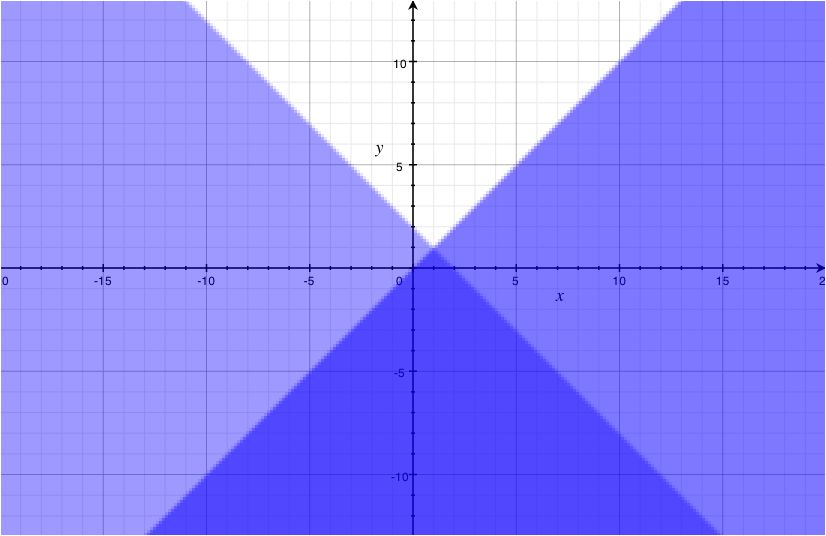Solve:
y > x y <− 2x
• Graph y > xb = 0m = 1
Use test point (1.0)
• Graph y <− 2xb = 0m = − 2
Use test point (1,0)
Graph both together to find the area of overlap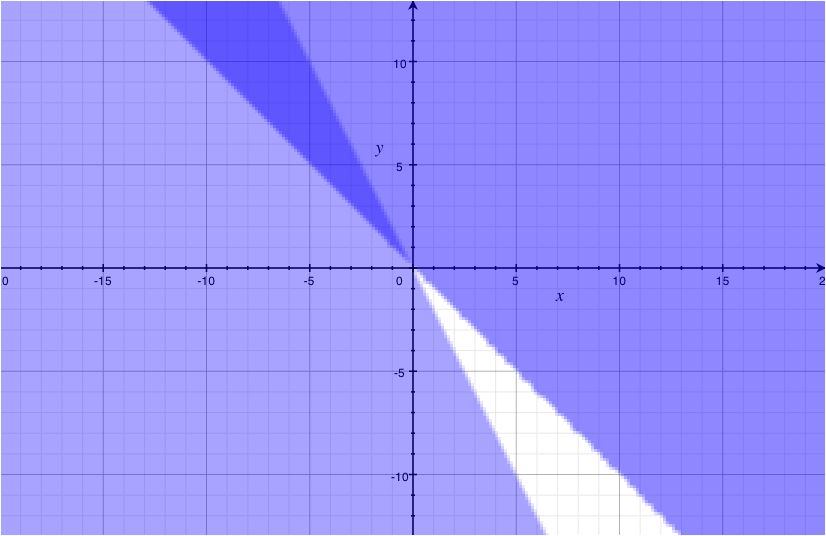Solve:
y > 3x + 1
y ≥ x − 1
• Graph y > 3x + 1b = 1m = 3
Use test point (0.0)
• Graph y ≥ x − 1b = − 1m = 1
Use test point (1,0)
Graph both together to find the area of overlap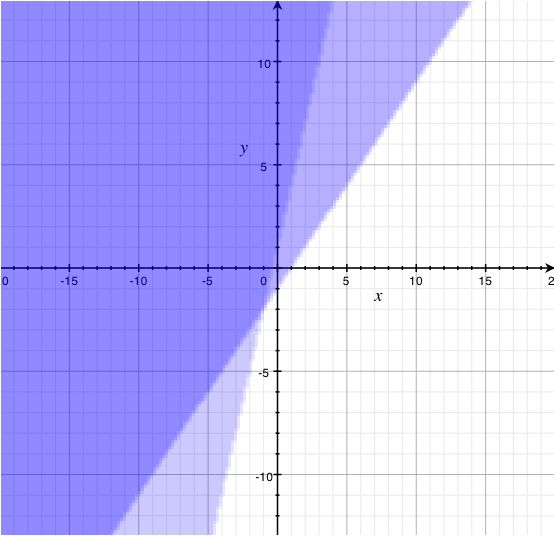Solve:
y ≤ 3
x ≥ 4
• Graph y ≤ 3
Horizontal line at y = 3
Use test point (0.0)
• Graph x ≥ 4
Vertical line at x = 4
Use test point (1,0)
Graph both together to find area of overlap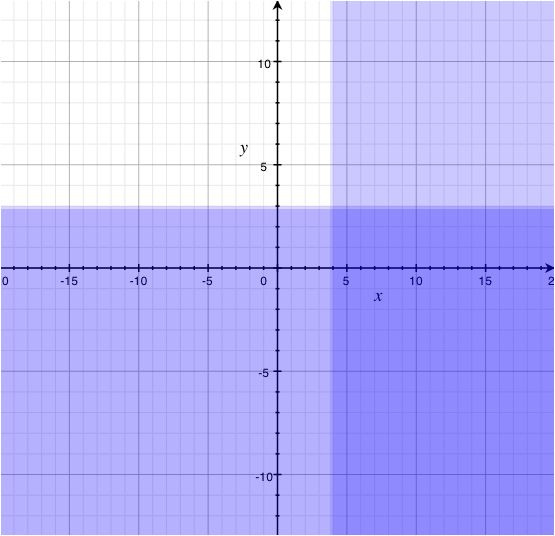Solve:
y ≤ [x/2] − 3
y < 5
• Graph y ≤ [x/2] − 3b = − 3m = [1/2]
Use test point (0.0)
• Graph y < 5
Horizontal line at y = 5
Use test point (1,0)
Graph both together to find area of overlap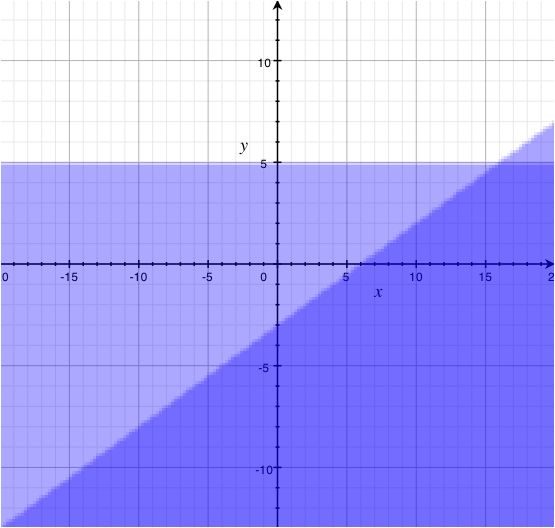Solve:
y ≤ 3x − 9
y ≥ − [x/3]
• Graph y ≤ 3x − 9b = − 9m = 3
Use test point (0.0)
• Graph y ≥ − [x/3]b = 0m = − [1/3]
Use test point (1,0)
Graph both together to find area of overlap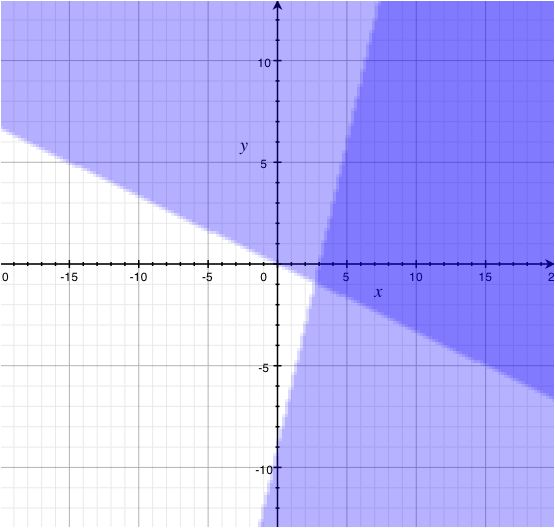Solve:
y ≤ − 4
y > 1
• Graph y ≤ − 4
Horizontal line at y = 4
Use test point (0.0)
• Graph y > 1
Horizontal line at y = 1
Use test point (1,0)
Graph both together to find area of overlap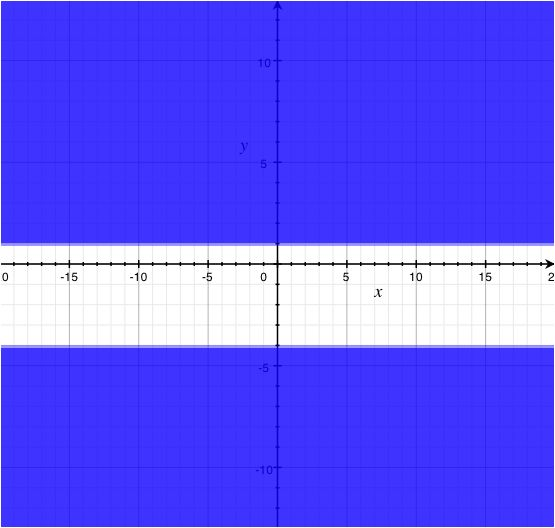No Solutions
Solve:
y > 4x − 2
y > x + 7
• Graph y > 4x - 2
b = - 2
m = 4
Use test point (0.0
• Graph y > x + 7
b = 7
m = 1
Use test point (1,0)
Graph both together to find area of overlap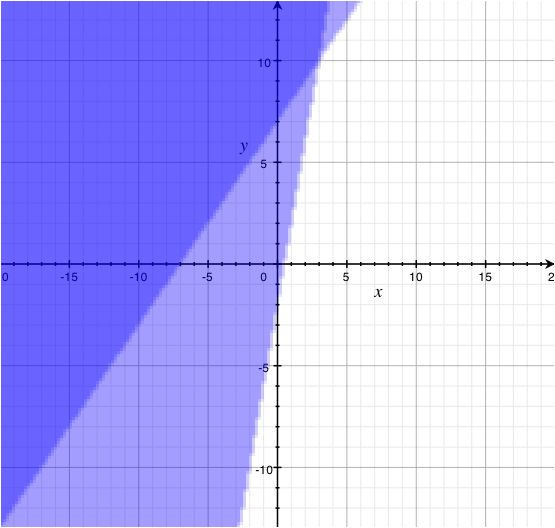Solve:
y + x < 7
2y + 2x >− 4
• Graph y + x < 7x = 0 → (0,7)y = 0 → (7,0)
Use test point (0.0)
• Graph 2y + 2x >− 4x = 0 → (0, − 2)y = 0 → ( − 2,0)
Use test point (0.0)
Graph both together to find area of overlap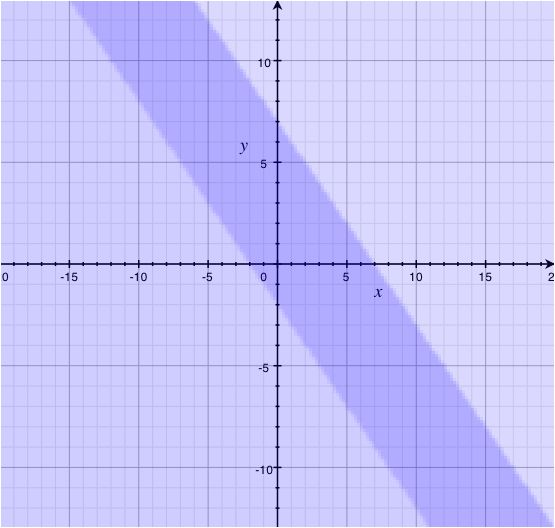Solve:
2y − 3x ≤ − 6
6y + 4x > 12
• Graph 2y − 3x ≤ − 6x = 0 → (0, − 3)y = 0 → (2,0)
Use test point (0.0)
• Graph 6y + 4x > 12x = 0(0,2)y = 0(3,0)
Use test point (0.0)
Graph both together to find area of overlap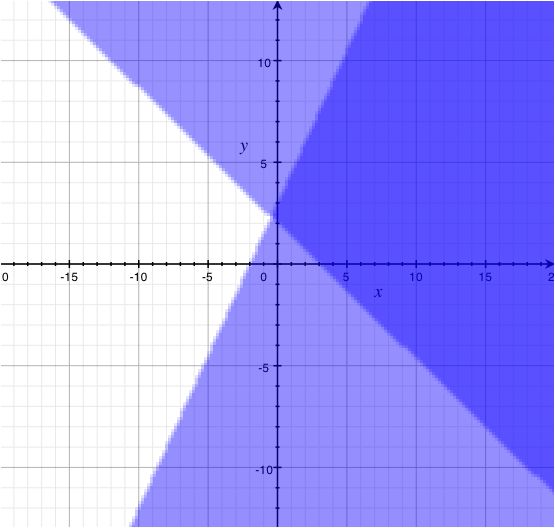*These practice questions are only helpful when you work on them offline on a piece of paper and then use the solution steps function to check your answer.

### Systems of Inequalities

Lecture Slides are screen-captured images of important points in the lecture. Students can download and print out these lecture slide images to do practice problems as well as take notes while watching the lecture.

• Intro 0:00
• Objectives 0:08
• Systems of Inequalities 0:24
• Test Points
• Steps to Solve Systems of Inequalities
• Example 1 2:23
• Example 2 7:28
• Example 3 12:51

### Transcription: Systems of Inequalities

Welcome back to www.educator.com.0000

In this lesson we are going to wrap things up with inequalities by looking at systems of inequalities.0002

We do not have a whole lot to cover but we will look at how you can satisfy a system of inequalities rather just individual inequalities.0011

That will bring us to looking at overlapping solution regions.0019

Recall it when we are usually dealing with the system, such as a system of equations,0027

we want to make sure that all of the equations in the system are satisfied.0031

When we are dealing with the system of inequalities, rather than equations, but it is the same goal.0037

We want to make sure that every single inequality in here gets satisfied.0042

This makes it a little unusual because rather than just looking for individual points that would satisfy inequality0048

remember that each of these is a region on our graph.0054

What we are looking for then is where these regions for each of them would end up overlapping.0059

That overlapping region would be where the entire system is satisfied.0065

Like before, after we are done identifying an overlapping region, we can use a test point to go ahead0070

and figure out whether that is the correct overlapping region.0077

It is not about process, we just have to get into figuring out what those regions are.0081

What we are going to do to basically figure out the overlapping region is, first we will graph each individual inequality in that system.0090

That breaks it down into figuring out the equation and figuring what side is shaded on that line.0099

Be very carefully that you are using either a dotted or dash line depending on the type of inequality in there.0107

We are using the dotted for our strict inequalities and we are using the nice solid line for our or equals to.0113

Since the line could be included as a solution.0122

Individually, we will make sure we shade on one side or the other and we can check that out using a test point.0126

Here is the big one that we have for our system, look for where the two regions overlap so we know where the solution to the system is.0132

That is a little bit of background, let us see these examples and see how this works in practice.0145

In my system of inequalities, I have 2x + y < or = 1 and I have x > 2y so individually let us take a look at these.0150

The corresponding equation to the first inequality would be 2x + y = 1.0161

If you want to graph that one out, you could get that one into slope intercept form by moving the 2x to the other side by subtracting a 2x.0169

From this I can see it has a y intercept of 1 and the slope of -2.0181

From that point I go down 2/1, down 2/1.0186

Looking at the original I can see it involves an or equals to so I'm going to use a nice solid line.0192

Now we have our equation, we need to figure out what side to shade it on.0212

We usually grab a test point to figure out what it should be and it is good to grab a nice test point that is to easy to evaluate.0216

I’m going to borrow the point 0, 0 here and see if it works in the original.0224

2x is 0, y is 0 is that less than or equal to 1, let us find out.0231

2 × 0 + = 0 and 0 < or = 1.0240

I know I should shade on that side of the line.0248

This entire region that we can see right now is only for the first inequality in our system.0255

Simply just going to go through the same process for the second inequality and figure out where both of their regions overlap.0265

Let us get some space and let us see this process for the second equation.0278

The second equation is x > 2y.0289

Maybe I will find this one in its slope intercept form so I will move the two together side by dividing everything by 2.0293

I could look at this as the equation y = ½ x, it has a y intercept of 0 and a slope of 1/2.0305

It goes through the origin 0 up 1/2, up 1/2, up 1/2, up ½.0317

Let us use a dotted line since the inequality is strict and see what we have.0325

We just need to shade on one side or the other.0348

We could test point for this particular line, let us just choose one way out here.0353

This one is at 3, 3.0358

Plug 3 in for y and plug 3 in for x and we will see if it is true not.0363

Is 3 < 3/2? That is not looking so good, that is not true.0376

What that means is we should actually shade on the other side of that line.0386

Let us shade everything below it.0390

Now comes the very important part of this.0402

We can see where each individual inequality is being satisfied but since we are interested in the region where the entire system is being satisfied,0406

we are looking at the overlapping regions.0418

I’m going to highlight that.0421

This region right down here is where the two overlap and I can see along one of the borders, it is dotted and along the other border it is solid.0424

This yellow area and its borders would be considered the solution to the system.0440

Let us try another of these and be careful as we walk through the process so we can see where that overlap is.0450

In this one we are dealing with the system 3x - 2y > 12 and 5x - y < 6.0455

Let us grab the first one and look at its corresponding equation.0463

The form of this ones in would be nice if we just use our intercepts to go ahead and see where it crosses the x and y axis.0477

I will put in a 0 for x and 0 for y.0486

If x = 0 what is y?0491

This term would go away for sure and I can divide both sides by -2 so y = -6.0496

Let us go ahead and put that on our graph, 0, -1, 2, 3, 4, 5, 6 right there.0507

We will go ahead and put in a 0 for y.0515

Let us see how this one turns out, 2 × 0 = 0, 3x = 12 divide both sides by 3 now and we will get x = 4.0524

4, 0 is our other point.0536

Now that we have 2 points, let us go ahead and connect it.0541

We will connect it using a dotted line and then we will figure out what side we should shade this on.0544

To determine what side to shade it on, let us borrow a nice, good test point.0564

Let us borrow the origin add 0, 0 and see if it is true or not.0569

(3 × 0) – (2 × 0) > 12? That would simplify to 0.0574

Is 0 > 12? No.0583

Let us shade the other side.0587

That entire side would be our solution region or least for the first one.0602

Let us get some space and do the same exact process for our second equation.0608

The corresponding equation would be 5x - y = 6.0622

This looks like maybe be easier to graph it if it was in slope intercept form.0629

I’m going to move the 5x to the other side and then multiply everything by -1.0634

Okay, my y intercept is way down there at -6 and it has a slope of 5 so up 1, 2, 3, 4, 5/1, up 1, 2, 3, 4, 5/1.0649

I will graph this one using a dotted line.0669

I have a few regions that it might be if we want to figure out until we shade one side of the blue line or the other.0692

Let us grab a test point for it.0701

I think origin 0, 0 is going to work out very nicely for this one as well.0702

(5 × 0) – 0 is that less than 6?0708

The entire left side simplifies to 0 and 0 < 6.0713

Let us go ahead and shade everything on that side of the line.0720

There is a lot of region over here to shade.0734

Now comes the important part where exactly do both of these overlap?0744

This is a little tough to tell, but if you look near the bottom you can see that there is the small triangle here where both of them overlap.0751

We would have that as our solution region.0761

As long as it is in the overlapping portion we are good to go.0765

For this last example I wanted something that was a little bit more like a word problem.0773

You could see how inequalities have a lot of good applications in them0777

and how some more problems lead to the rise of a system of inequalities rather than a system of equations.0781

What we want to do for this one is graph the solution region for the system and interpret what that means in the context of the problem.0789

What we are looking to do is recreate what it means when a person's heart rate,0797

or a person's maximum heart rate 220 – x, where x is the person’s age.0802

This one is good as long as it is for people between 20 and 70 years old.0808

When a person exercises and are trying to lose some weight, it is recommended that the person strive for heart rate0815

that is at least 60% of the maximum and at most 70% of the maximum.0820

There is a little bit of a range for that maximum heart rate that we are aiming for.0826

Let us see if we can interpret some of the things in here and create an inequality for what we have.0832

First of all, let us talk about a person's age.0839

Earlier it says that x is our person's age and that we are only interested when x is in between 20 and 70.0844

Let us say x > 20 and x < 70.0856

There are two inequalities that we can put in our system.0864

We also want to look at a person losing weight.0868

Also, it recommends that they strive for 60% of their maximum and no more than 70% of their maximum.0873

What exactly is their maximum?0880

It is way up here at this expression, 220 - x.0883

60% of their maximum 3.60 (220 – x) and we want to make sure their heart rate is greater than that.0890

You do not want to stress them too much, so we will make sure that it is less than .70 of the maximum heart rate.0915

What we now have here are 1, 2, 3, 4 different inequalities in our system that we will be looking to satisfy.0924

And we will graph this out so we can see the region it creates.0934

Let us end up rewriting this just so we have them handy.0943

x must be greater than 20, x is less than 70,0947

and we also have two more, H is greater than (.6 × 220) - x and H is less than (.7 × 220) – x.0955

If I'm going to graph these other two here I want to get them in a better form.0974

It is tough to see maybe what their slope is and what their y intercepts is.0980

Let us do a little bit of work with distributing that .6 and .7 in so we have a better idea.0985

This one will be H > .6 × 220 = 132 - .6x and for this other one we have 154 -.7x, that gives us a much better idea.0995

I think I’m going to write them again so I can put that x term first and see that is my slope.1020

We will end up graphing these two inequalities in our system as well as these two on our system.1039

Let us see how we can do that.1046

With the first two that have to deal with the age of the person, we will use that along our x axis.1048

We want the age of the person to be between 20 and 70.1059

Let us use a nice vertical line and I’m going to make this dotted because my inequality is strict.1068

Let us do the same thing for our 70 here.1080

If I was looking to just satisfy those first two then I would have to be between of these two lines.1092

Let us work in what we want their heart rate to be and see how that fits into this.1104

In the first one I can see it has a y intercept of 132 and the other one has a y intercept of 154.1113

My slope on the first one is a -.6x so since it is negative it should be going down, maybe something like this.1127

In the other one is very similar its slope is negative as well, but it is a little bit steeper since its .7 and we will put that on.1153

Now where should the shading for this one be?1174

We want our heart rate to be more than the .6x + 132.1177

We want to think of that as our minimum heart rate that we are aiming for in terms of exercise.1183

The other one would be a .7x + 157 we want them to be no greater than that value.1189

We are looking in between these 2 lines again.1195

From looking at these two solution regions, it is this little box looking thing where they end up overlapping.1202

We can interpret what that solution region means in terms of the problem.1216

If you we are to just pick a random point inside that box, what we are getting is maybe an age of a person,1221

let us say 65 and we are also getting what the heart rate should be when they exercise to try and lose weight.1231

As long as they stay inside that box they should do some good things to their heart.1239

If we try and fall outside of the solution region that means either the formula is for our maximum heart rate no longer apply1245

or we would end up overtaxing the heart and either one we definitely do not want.1254

When setting up a system of inequalities look at them individually and graph out each of the regions.1261

Then look at the overlap and see what would be included in both.1267

Thank you for watching www.educator.com.1271

OR

### Start Learning Now

Our free lessons will get you started (Adobe Flash® required).